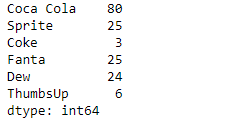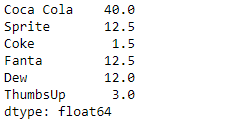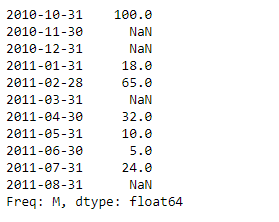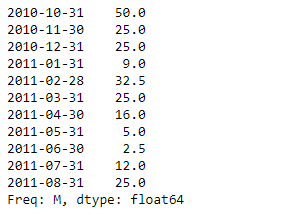Related Articles

# Python | Pandas Series.divide()

• Last Updated : 15 Feb, 2019

Pandas series is a One-dimensional ndarray with axis labels. The labels need not be unique but must be a hashable type. The object supports both integer- and label-based indexing and provides a host of methods for performing operations involving the index.

Pandas` Series.divide()` function performs floating division of series and other, element-wise (binary operator truediv). It is equivalent to `series / other`, but with support to substitute a fill_value for missing data in one of the inputs.

Syntax: Series.divide(other, level=None, fill_value=None, axis=0)

Parameter :
other : Series or scalar value
fill_value : Fill existing missing (NaN) values.
level : Broadcast across a level, matching Index values on the passed MultiIndex level

Returns : result : Series

Example #1: Use `Series.divide()` function to perform floating division of the given series object with a scalar.

 `# importing pandas as pd``import` `pandas as pd`` ` `# Creating the Series``sr ``=` `pd.Series([``80``, ``25``, ``3``, ``25``, ``24``, ``6``])`` ` `# Create the Index``index_ ``=` `[``'Coca Cola'``, ``'Sprite'``, ``'Coke'``, ``'Fanta'``, ``'Dew'``, ``'ThumbsUp'``]`` ` `# set the index``sr.index ``=` `index_`` ` `# Print the series``print``(sr)`

Output :Now we will use `Series.divide()` function to perform floating division of the given series object with a scalar.

 `# perform floating division``result ``=` `sr.divide(other ``=` `2``)`` ` `# Print the result``print``(result)`

Output :As we can see in the output, the `Series.divide()` function has successfully performed the floating division of the given series object with a scalar.

Example #2 : Use `Series.divide()` function to perform floating division of the given series object with a scalar. The given series object contains some missing values.

 `# importing pandas as pd``import` `pandas as pd`` ` `# Creating the Series``sr ``=` `pd.Series([``100``, ``None``, ``None``, ``18``, ``65``, ``None``, ``32``, ``10``, ``5``, ``24``, ``None``])`` ` `# Create the Index``index_ ``=` `pd.date_range(``'2010-10-09'``, periods ``=` `11``, freq ``=``'M'``)`` ` `# set the index``sr.index ``=` `index_`` ` `# Print the series``print``(sr)`

Output :Now we will use `Series.divide()` function to perform floating division of the given series object with a scalar. We are going to fill 50 at the place of all the missing values.

 `# perform floating division``# fill 50 at the place of missing values``result ``=` `sr.divide(other ``=` `2``, fill_value ``=` `50``)`` ` `# Print the result``print``(result)`

Output :As we can see in the output, the `Series.divide()` function has successfully performed the floating division of the given series object with a scalar.

Attention geek! Strengthen your foundations with the Python Programming Foundation Course and learn the basics.

To begin with, your interview preparations Enhance your Data Structures concepts with the Python DS Course. And to begin with your Machine Learning Journey, join the Machine Learning – Basic Level Course

My Personal Notes arrow_drop_up